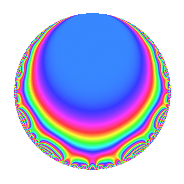# Properties

 Label 71.1.bLevel 71 Weight 1 Character orbit b Rep. character $$\chi_{71}(70,\cdot)$$ Character field $$\Q$$ Dimension 3 Newforms 1 Sturm bound 6 Trace bound 0

# Related objects

## Defining parameters

 Level: $$N$$ = $$71$$ Weight: $$k$$ = $$1$$ Character orbit: $$[\chi]$$ = 71.b (of order $$2$$ and degree $$1$$) Character conductor: $$\operatorname{cond}(\chi)$$ = $$71$$ Character field: $$\Q$$ Newforms: $$1$$ Sturm bound: $$6$$ Trace bound: $$0$$

## Dimensions

The following table gives the dimensions of various subspaces of $$M_{1}(71, [\chi])$$.

Total New Old
Modular forms 4 4 0
Cusp forms 3 3 0
Eisenstein series 1 1 0

The following table gives the dimensions of subspaces with specified projective image type.

$$D_n$$ $$A_4$$ $$S_4$$ $$A_5$$
Dimension 3 0 0 0

## Trace form

 $$3q$$ $$\mathstrut -\mathstrut q^{2}$$ $$\mathstrut -\mathstrut q^{3}$$ $$\mathstrut +\mathstrut 2q^{4}$$ $$\mathstrut -\mathstrut q^{5}$$ $$\mathstrut -\mathstrut 2q^{6}$$ $$\mathstrut -\mathstrut 2q^{8}$$ $$\mathstrut +\mathstrut 2q^{9}$$ $$\mathstrut +\mathstrut O(q^{10})$$ $$3q$$ $$\mathstrut -\mathstrut q^{2}$$ $$\mathstrut -\mathstrut q^{3}$$ $$\mathstrut +\mathstrut 2q^{4}$$ $$\mathstrut -\mathstrut q^{5}$$ $$\mathstrut -\mathstrut 2q^{6}$$ $$\mathstrut -\mathstrut 2q^{8}$$ $$\mathstrut +\mathstrut 2q^{9}$$ $$\mathstrut -\mathstrut 2q^{10}$$ $$\mathstrut -\mathstrut 3q^{12}$$ $$\mathstrut -\mathstrut 2q^{15}$$ $$\mathstrut +\mathstrut q^{16}$$ $$\mathstrut +\mathstrut 4q^{18}$$ $$\mathstrut -\mathstrut q^{19}$$ $$\mathstrut +\mathstrut 4q^{20}$$ $$\mathstrut +\mathstrut 3q^{24}$$ $$\mathstrut +\mathstrut 2q^{25}$$ $$\mathstrut -\mathstrut 2q^{27}$$ $$\mathstrut -\mathstrut q^{29}$$ $$\mathstrut +\mathstrut 3q^{30}$$ $$\mathstrut -\mathstrut 3q^{32}$$ $$\mathstrut -\mathstrut q^{36}$$ $$\mathstrut -\mathstrut q^{37}$$ $$\mathstrut +\mathstrut 5q^{38}$$ $$\mathstrut -\mathstrut 4q^{40}$$ $$\mathstrut -\mathstrut q^{43}$$ $$\mathstrut -\mathstrut 3q^{45}$$ $$\mathstrut +\mathstrut 2q^{48}$$ $$\mathstrut +\mathstrut 3q^{49}$$ $$\mathstrut -\mathstrut 3q^{50}$$ $$\mathstrut -\mathstrut 4q^{54}$$ $$\mathstrut -\mathstrut 2q^{57}$$ $$\mathstrut -\mathstrut 2q^{58}$$ $$\mathstrut +\mathstrut q^{60}$$ $$\mathstrut +\mathstrut 3q^{71}$$ $$\mathstrut +\mathstrut q^{72}$$ $$\mathstrut -\mathstrut q^{73}$$ $$\mathstrut +\mathstrut 5q^{74}$$ $$\mathstrut +\mathstrut 4q^{75}$$ $$\mathstrut -\mathstrut 3q^{76}$$ $$\mathstrut -\mathstrut q^{79}$$ $$\mathstrut +\mathstrut 2q^{80}$$ $$\mathstrut +\mathstrut q^{81}$$ $$\mathstrut -\mathstrut q^{83}$$ $$\mathstrut -\mathstrut 2q^{86}$$ $$\mathstrut +\mathstrut 5q^{87}$$ $$\mathstrut -\mathstrut q^{89}$$ $$\mathstrut +\mathstrut q^{90}$$ $$\mathstrut -\mathstrut 2q^{95}$$ $$\mathstrut +\mathstrut q^{96}$$ $$\mathstrut -\mathstrut q^{98}$$ $$\mathstrut +\mathstrut O(q^{100})$$

## Decomposition of $$S_{1}^{\mathrm{new}}(71, [\chi])$$ into irreducible Hecke orbits

Label Dim. $$A$$ Field Image CM RM Traces $q$-expansion
$$a_2$$ $$a_3$$ $$a_5$$ $$a_7$$
71.1.b.a $$3$$ $$0.035$$ $$\Q(\zeta_{14})^+$$ $$D_{7}$$ $$\Q(\sqrt{-71})$$ None $$-1$$ $$-1$$ $$-1$$ $$0$$ $$q-\beta _{1}q^{2}+(-1+\beta _{1}-\beta _{2})q^{3}+(1+\beta _{2})q^{4}+\cdots$$